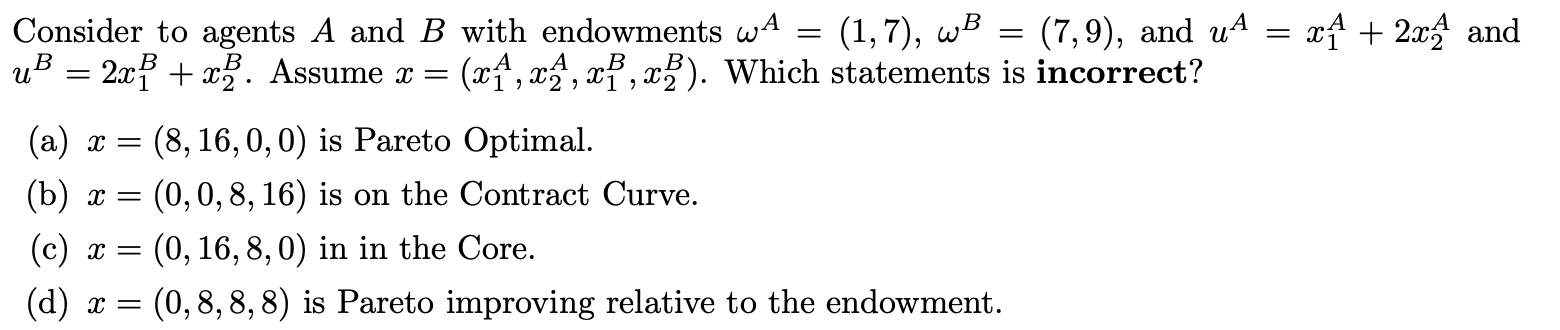### Create an Account

Already have account?

### Forgot Your Password ?

Home / Questions / x+ 2x4 and Consider to agents A and B with endowments wa (1,7), WB (7,9), and us uB = 2x +...

# x+ 2x4 and Consider to agents A and B with endowments wa (1,7), WB (7,9), and us uB = 2x + x). Assume x = (xA, XA, XP,

= = x+ 2x4 and Consider to agents A and B with endowments wa (1,7), WB (7,9), and us uB = 2x + x). Assume x = (xA, XA, XP,Apr 19 2021 View more View Less

#### Answer (Solved)Subscribe To Get Solution